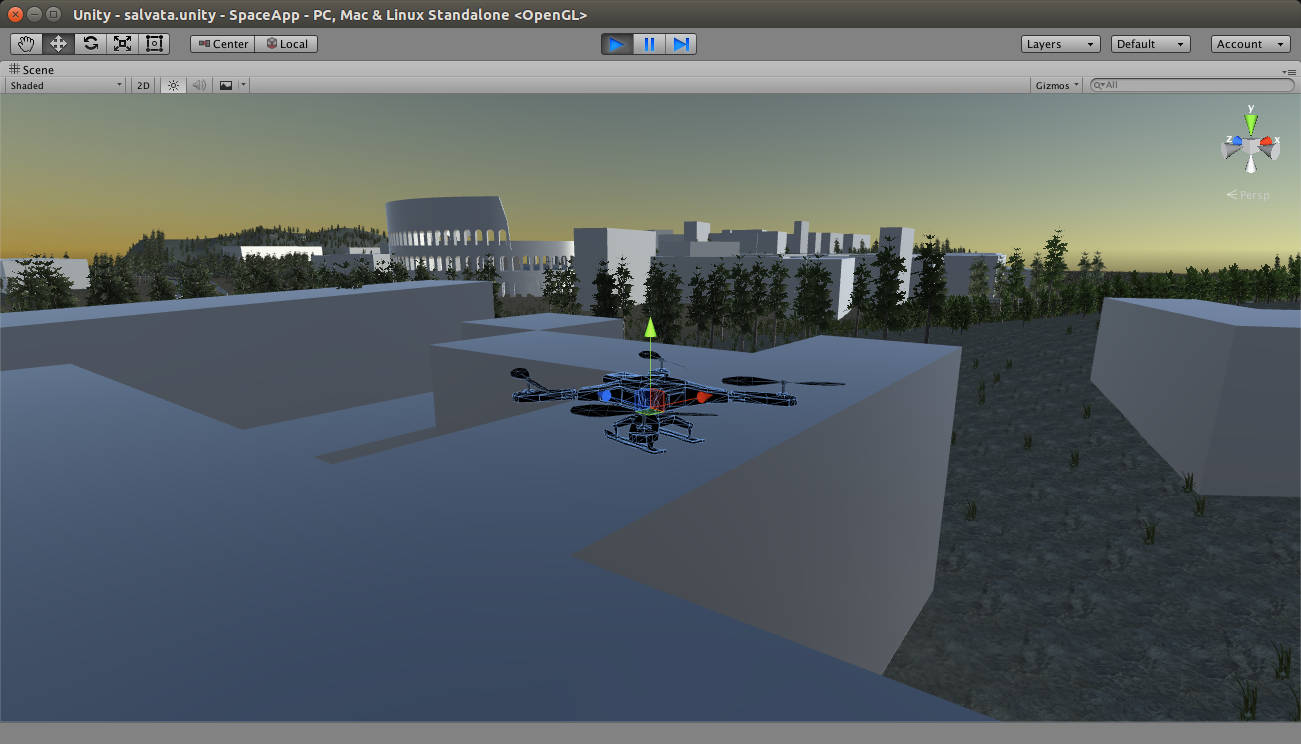# trajectory

•## Python – Scikits Candidate Optimal trajectory

|

On the last article we have installed the Python Scikits library and run the samples provided. In this quick article we would like to find a candidate optimal trajectory that minimizes a weighted combination of speed and position values during a considered time interval $t_0=0$ s and $t_f=10$s. The performance measure $J(u)$ looks like

•## Python – Scikits Two Point Boundary Value Problem

|

An application of Calculus of Variation is the determination of control action $u(t)$ such that a system described by a differential equation system $$\dot{x}(t) = {a(x(t),u(t),t)}.$$ follows a trajectory $x(t)$ for all time instants that minimizes a performance measure $J(u)$ defined by $$J(u) = \int_{t0}^{tf} {g(x(t),u(t),t)} dt$$ A wonderful application could be a tridimentional trajectory